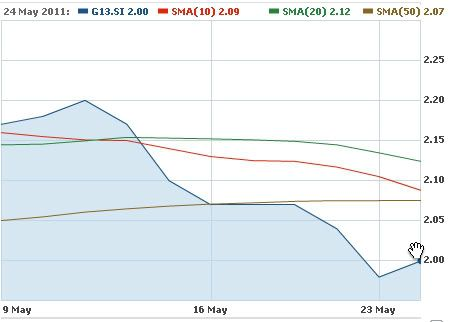# Stock Moving Average Calculation

Moving Averages can help traders to measure the direction of the trend. Most of the time technical analysts are likely to use 10 and 50-day moving averages. It focuses on the average price which is set based on a predefined time range. If you want to calculate the moving average of a trend, then you need to keep track the historical price.

How to Calculate a Moving Average?

It is very simple to calculate the Moving Average. Let say you want 10 day Moving Average, then you just simply add all 10 days prices, and divide the sum by 10 (length of day).

Example:

The stock prices of Genting Singapore PLC for past 10 days are as below:
2.18, 2.20, 2.17, 2.10, 2.07, 2.07, 2.07, 2.04, 1.98, 2.00

Calculation for (10-day MA) = (2.18, 2.20, 2.17, 2.10, 2.07, 2.07, 2.07, 2.04, 1.98, 2.00)/10 = 2.08.

If the trader wishes to calculate a 50-day MA, then he just need get the set of prices for last 50 days to compute the result.